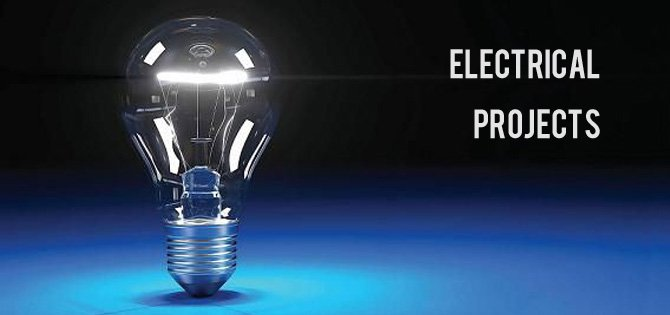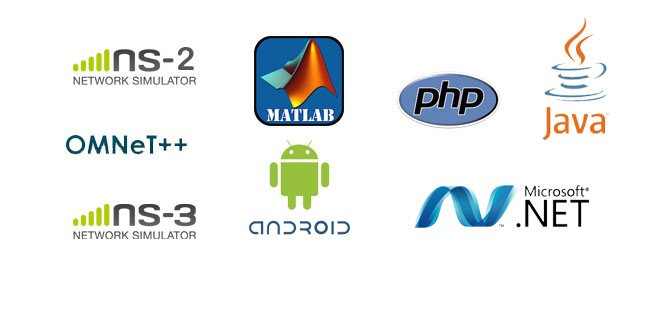•••We provide electrical projects based on power electronics, MATLAB Simulink and SIM Power

•For Electronics Engineering Students we support technologies like ARM, GSM, GPS, RFID, Robotics, VLSI, NSL, NS3, OMNet++, OPNet, QUALNET, PeerSim

•For Computer Science Students we support NS2, NS3, MATLAB, Java, OMNeT++, OPNet, CloudSim, Qualnet, Weka, Wordnet, Dot Net, Hadoop,….

# MATLAB Projects For Engineering Students

MATLAB Projects For Engineering Students helps to the computer science ,networking , electrical students to develop their projects.MATLAB  is an important simulation tool used in various domains for final year projects. We offer final year project matlab with mathematical and functional algorithm to develop surveillance, security system, and digital image processing, biomedical image processing and information security from ACM papers. We support B.E electrical and communication engineering, computer science engineering, information technology students to do their final year project in matlab. We implement brain segmentation, human ear recognition, identifying human activities, lung segmentation, breast cancer MRI images, real time facial expression recognition and contact lens on iris recognition under final year projects in matlab. We developed more than 80+ project in matlab with various techniques.

## Projects on Matlab:

Engineering students are interestingly to choose their final year academic projects on image processing domain. Image processing has a few sub domains. There are digital image processing, biomedical engineering, signal processing, medical imaging and remote sensing. In digital image processing Image analysis and image transformation processes are easily simulated based on matlab simulation. Remote sensing concepts are used to extract lineament features, underwater sea object detection, shadow detection and fused changes detection. Speech recognition concepts are comes under signal processing. Several speech recognition techniques are used to generate signals and echo cancellation process. Biomedical engineering concepts are briefly described below.

## Bio medical image reconstruction by matlab:

We process various image reconstructions in biomedical science we provide advanced and computation demand reconstruction algorithm which efficiently reconstruct medical image. We introduce various sampling method in final year matlab project by project developer for magnetic resonance imaging. We permit conjugate gradient, least square QR decomposition method, minimum residual and complex orthogonal conjugate gradient in matlab to solver linear problem in bio medical image classification method.

## Hybrid multi view stereo algorithm for modeling urban scenes:

We propose stereo reconstruction algorithm in final year matlab projects. By this algorithm we create 3D model of urban image by joining mesh and geometric primitives. This algorithm provide compact model to preserve irregular elements and regular structures. We segment initial mesh based surface using a multi label markov random file based model. We determine jump diffusion process to extract sampling primitives and mesh components in a same time. We use matlab to qualify reconstruction measures and simulate multi objectives energy model of photo consistency and semantic consideration. We determine iterative refinement procedure to ensure accurate hybrid representation than other view stereo meshing algorithm.

## Estimate intrinsic image from photograph:

We provide photographic images with significant user intervention, reflection and illumination variation, multiple images under varying lightening. We introduce inverse global illumination method in photographic based matlab projects which eradicate material scene properties but still it require high accurate geometrical model for inverse rendering, image based propagation algorithm, intrinsic image and patch based multi view stereo algorithm to extract intrinsic image from photographic images.

## Discriminative Gabor feature selection:

We introduce 3D Gabor wavelet method for hyper spectral image classification. By Gabor filter we extract features as spatial & spectrum information from large scale images. We perform process by symmetrical uncertain & markov blank let based approach.

## Quantitative evolution of transform domain by sampling based recovery:

We provide volumetric image by comprehensive sampling method to significantly show image volumes. We used multidimensional wavelet transform to recover image volume. We determine 3D fast Fourier transformation, surface let, discrete cosine transformation to reconstruct optical coherence tomography image from sub sampled image dataset. We evaluate image quality, volume & Differ transform by structural similarity index and mean square error.

MATLAB Projects For Engineering Students